# 小白也能看懂的 ROC 曲线详解

07/21 10:13ROC 曲线是一种坐标图式的分析工具，是由二战中的电子和雷达工程师发明的，发明之初是用来侦测敌军飞机、船舰，后来被应用于医学、生物学、犯罪心理学。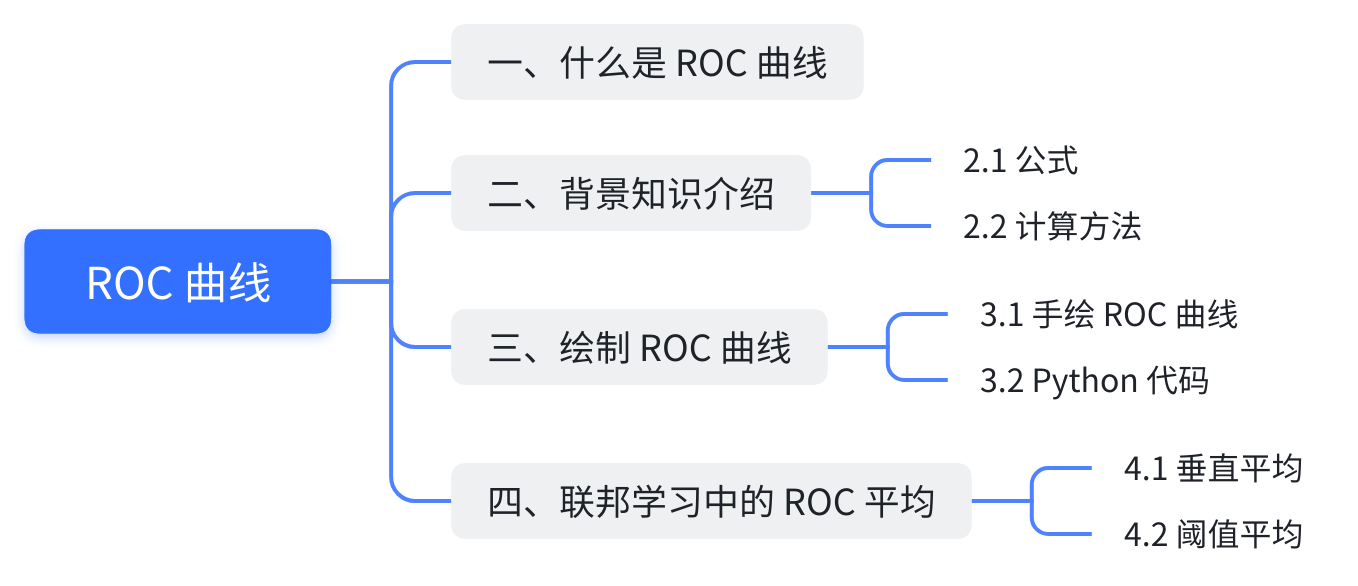## 一、什么是 ROC 曲线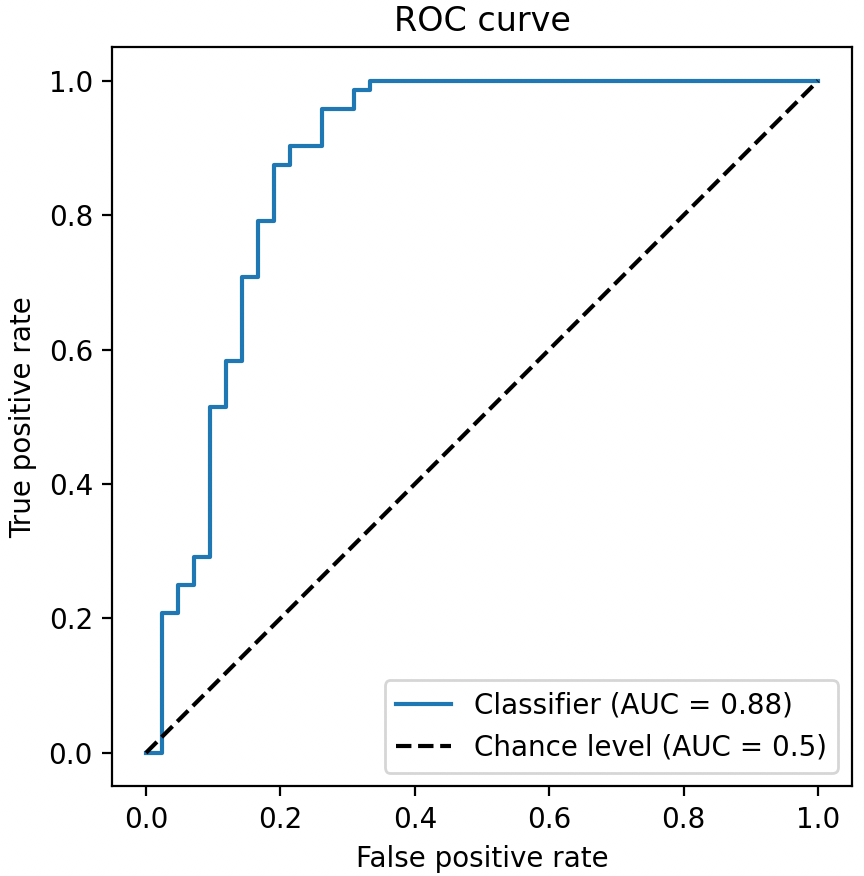• ROC 曲线接近左上角：模型预测准确率很高
• ROC 曲线略高于基准线：模型预测准确率一般
• ROC 低于基准线：模型未达到最低标准，无法使用

## 二、背景知识

• 标签 $y$ 的取值为 0 或 1。
• 模型预测的标签为 $\hat{y}$，取值也是 0 或 1。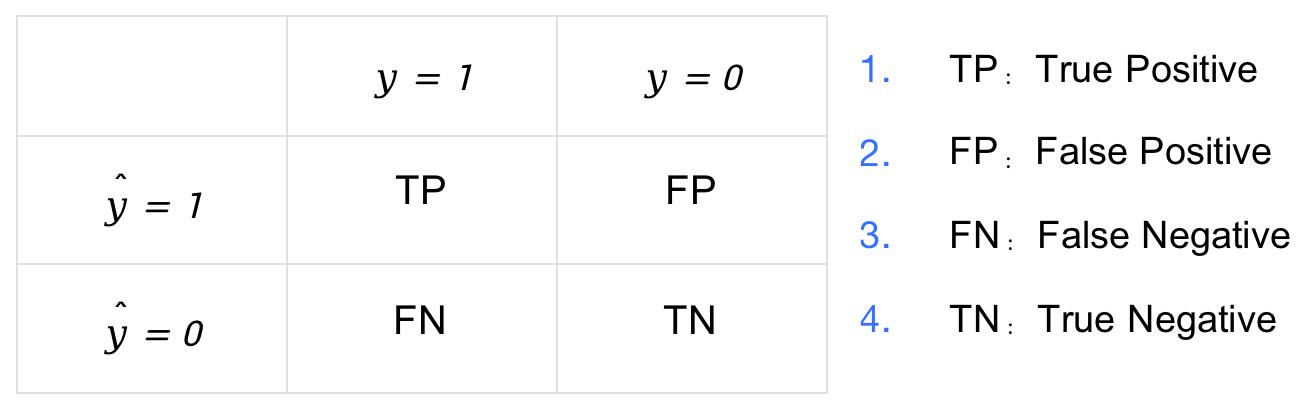### 2.1 公式

ROC 曲线的横坐标为 FPR（False Positive Rate），纵坐标为 TPR（True Positive Rate）。FPR 统计了所有负样本中 预测错误(FP) 的比例，TPR 统计了所有正样本中 预测正确(TP) 的比例，其计算公式如下，其中 # 表示统计个数，例如 #N 表示负样本的个数，#P 表示正样本的个数

$\text{FPR}=\frac{\#\text{FP}}{\#\text{N}}$$\text{TPR}=\frac{\#\text{TP}}{\#\text{P}}$

### 2.2 计算方法

id 真实标签$y$ 预测标签$\hat{y}$
1 1 1
2 1 0
3 0 0
4 1 1
5 0 1

FPR 和 TPR 的取值范围均是 0 到 1 之间。对于 FPR，我们希望其越小越好。而对于 TPR，我们希望其越大越好。

## 三、绘制 ROC 曲线

• 第一部分：通过手绘的方式讲解原理
• 第二部分：Python 代码实现，代码清爽易读

### 3.1 手绘 ROC 曲线

id 真实标签 预测分数 id 真实标签 预测分数
1 1 .9 11 1 .4
2 1 .8 12 0 .39
3 0 .7 13 1 .38
4 1 .6 14 0 .37
5 1 .55 15 0 .36
6 1 .54 16 0 .35
7 0 .53 17 1 .34
8 0 .52 18 0 .33
9 1 .51 19 1 .30
10 0 .505 20 0 .1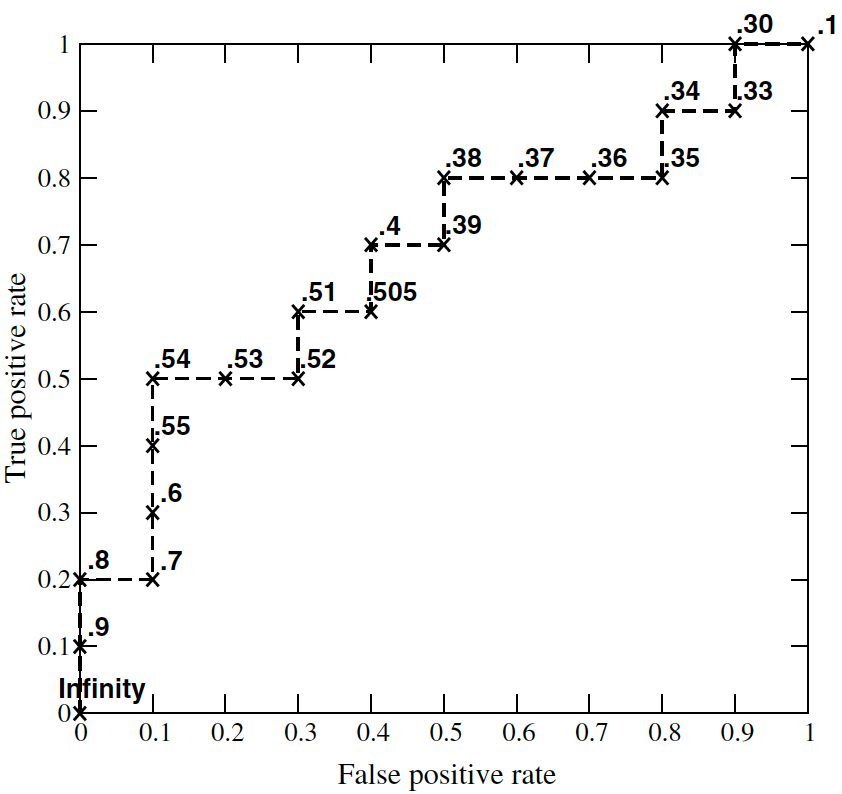### 3.2 Python 代码# import numpy as np
def roc(y_true, y_score, pos_label):
"""
y_true：真实标签
y_score：模型预测分数
pos_label：正样本标签，如“1”
"""
# 统计正样本和负样本的个数
num_positive_examples = (y_true == pos_label).sum()
num_negtive_examples = len(y_true) - num_positive_examples

tp, fp = 0, 0
tpr, fpr, thresholds = [], [], []
score = max(y_score) + 1

# 根据排序后的预测分数分别计算fpr和tpr
for i in np.flip(np.argsort(y_score)):
# 处理样本预测分数相同的情况
if y_score[i] != score:
fpr.append(fp / num_negtive_examples)
tpr.append(tp / num_positive_examples)
thresholds.append(score)
score = y_score[i]

if y_true[i] == pos_label:
tp += 1
else:
fp += 1

fpr.append(fp / num_negtive_examples)
tpr.append(tp / num_positive_examples)
thresholds.append(score)

return fpr, tpr, thresholds


import numpy as np

y_true = np.array(
[1, 1, 0, 1, 1, 1, 0, 0, 1, 0, 1, 0, 1, 0, 0, 0, 1, 0, 1, 0]
)
y_score = np.array([
.9, .8, .7, .6, .55, .54, .53, .52, .51, .505,
.4, .39, .38, .37, .36, .35, .34, .33, .3, .1
])

fpr, tpr, thresholds = roc(y_true, y_score, pos_label=1)


import matplotlib.pyplot as plt

plt.plot(fpr, tpr)
plt.axis("square")
plt.xlabel("False positive rate")
plt.ylabel("True positive rate")
plt.title("ROC curve")
plt.show()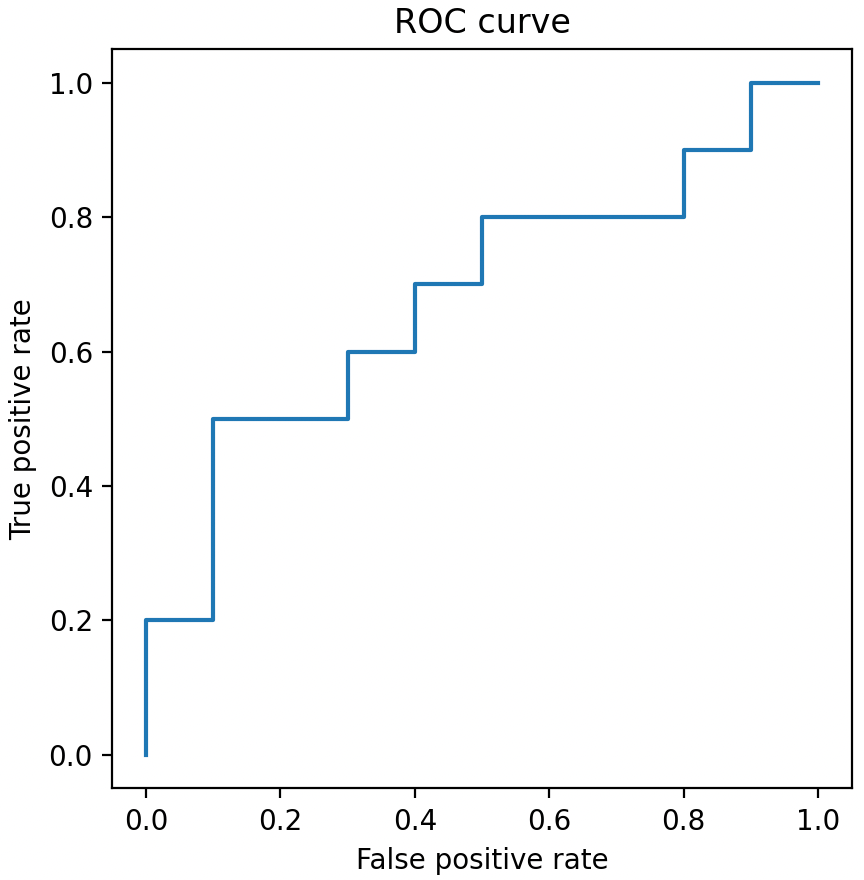## 四、联邦学习中的 ROC 平均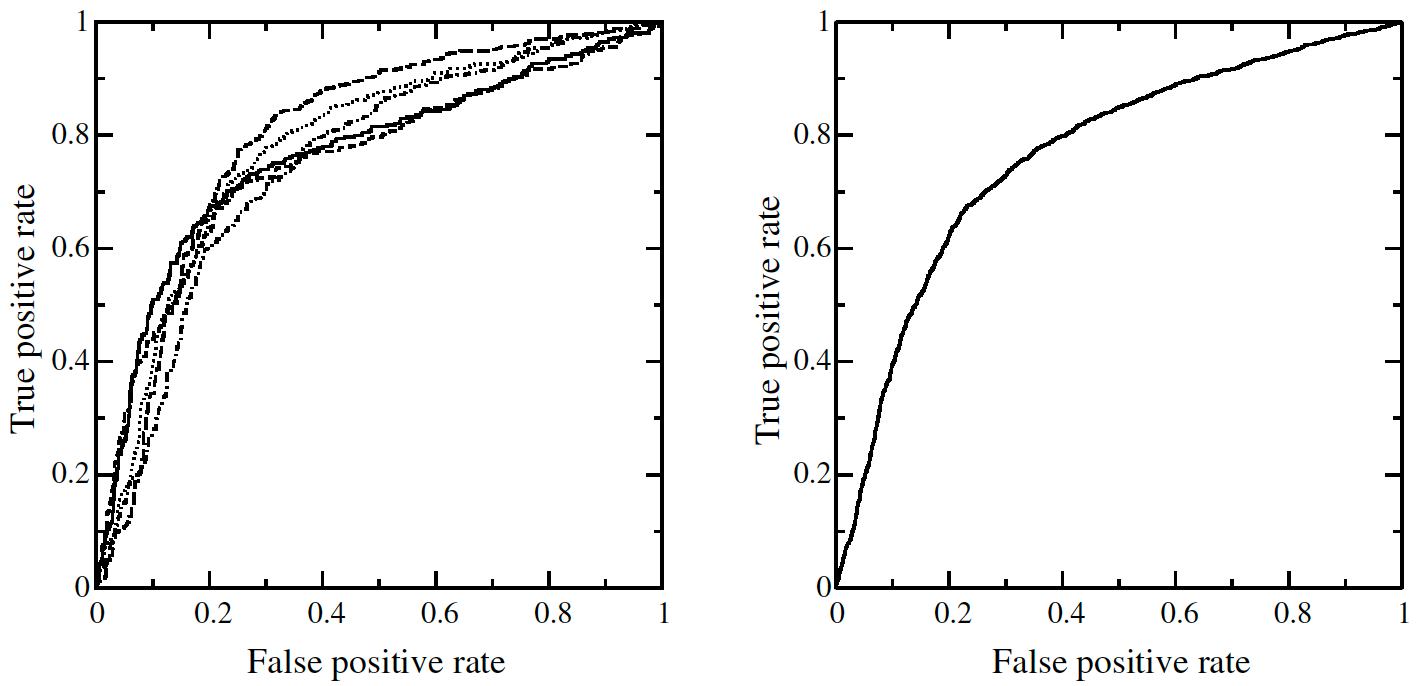ROC 的平均有两种方法：垂直平均、阈值平均，下面将逐一进行讲解，并给出 Python 代码实现。

### 4.1 垂直平均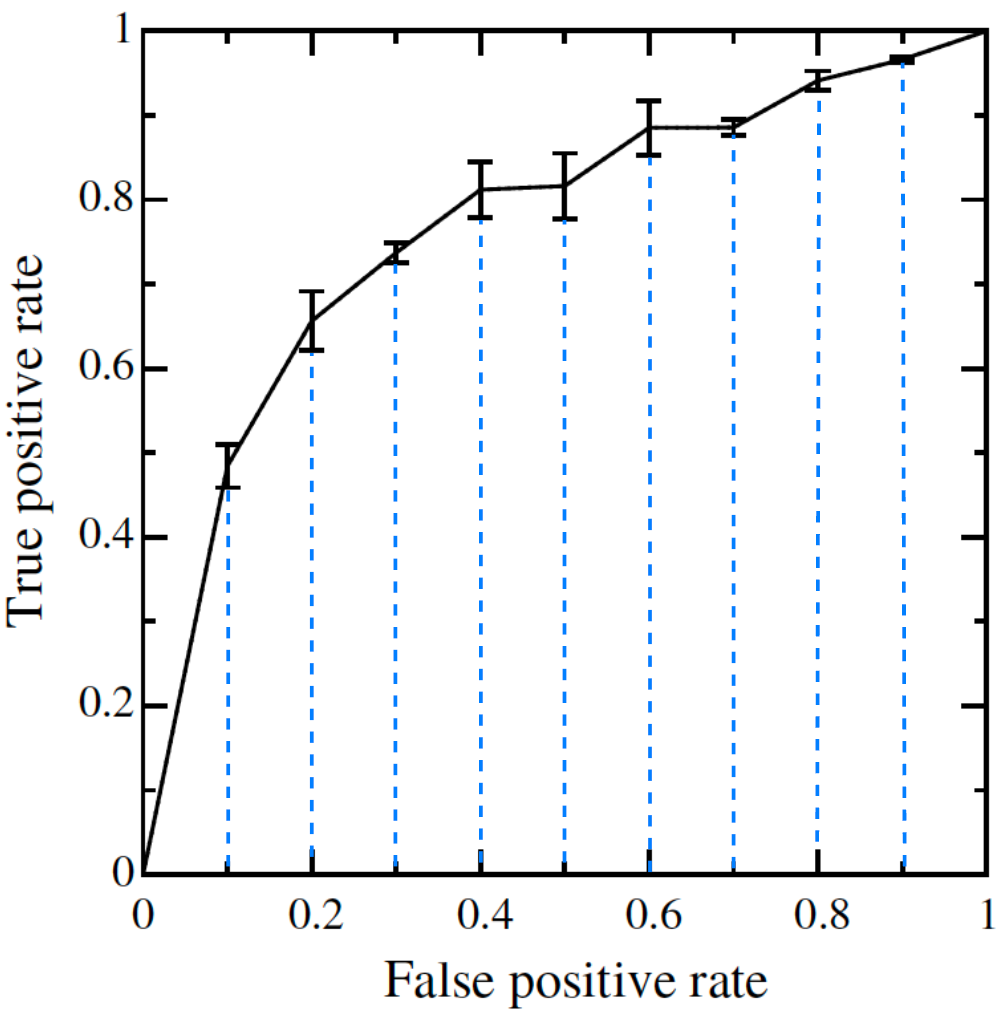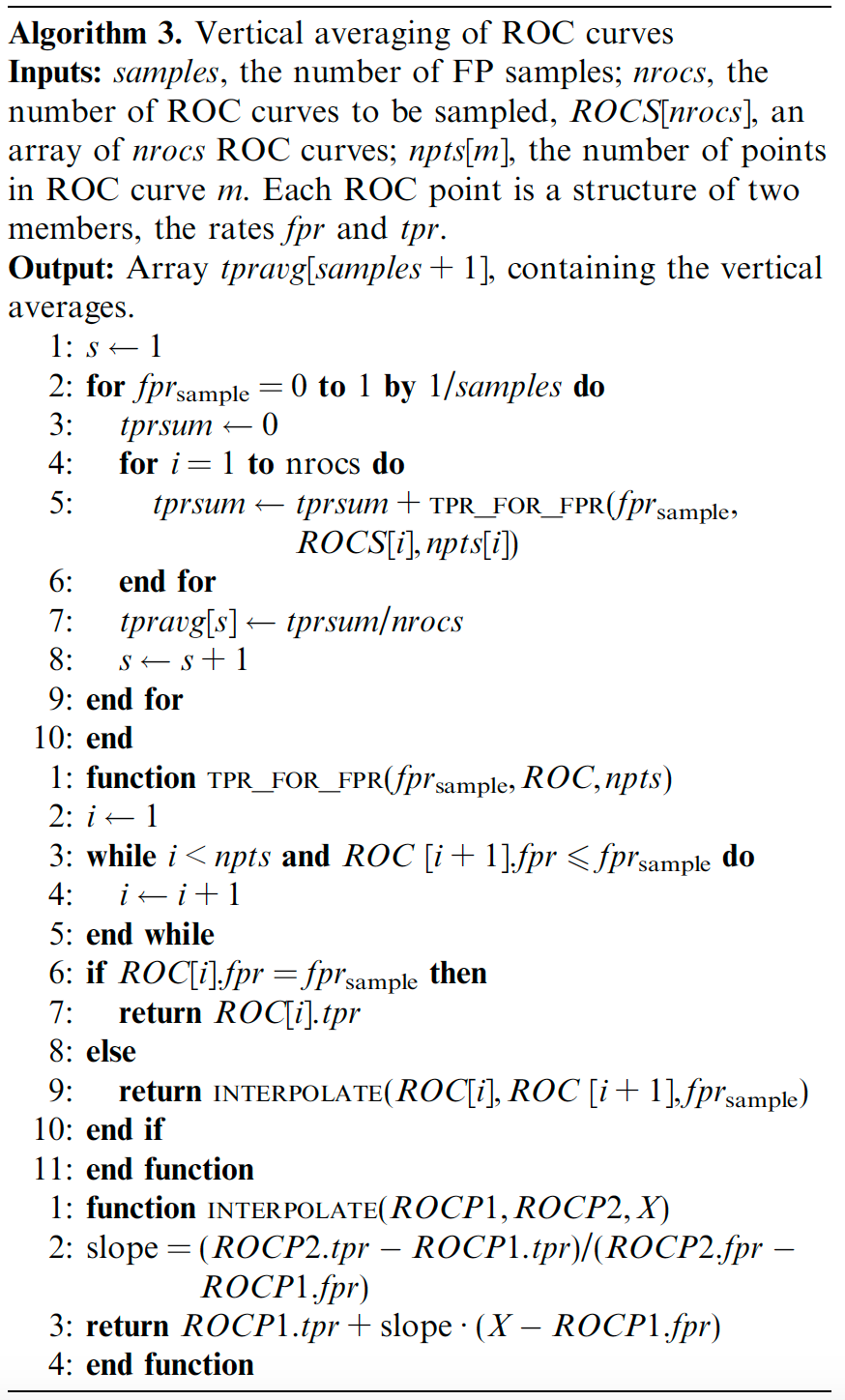# import numpy as np
def roc_vertical_avg(samples, FPR, TPR):
"""
samples：选取FPR点的个数
FPR：包含所有FPR的列表
TPR：包含所有TPR的列表
"""
nrocs = len(FPR)
tpravg = []
fpr = [i / samples for i in range(samples + 1)]

for fpr_sample in fpr:
tprsum = 0
# 将所有计算的tpr累加
for i in range(nrocs):
tprsum += tpr_for_fpr(fpr_sample, FPR[i], TPR[i])
# 计算平均的tpr
tpravg.append(tprsum / nrocs)

return fpr, tpravg

# 计算对应fpr的tpr
def tpr_for_fpr(fpr_sample, fpr, tpr):
i = 0
while i < len(fpr) - 1 and fpr[i + 1] <= fpr_sample:
i += 1

if fpr[i] == fpr_sample:
return tpr[i]
else:
return interpolate(fpr[i], tpr[i], fpr[i + 1], tpr[i + 1], fpr_sample)

# 插值
def interpolate(fprp1, tprp1, fprp2, tprp2, x):
slope = (tprp2 - tprp1) / (fprp2 - fprp1)
return tprp1 + slope * (x - fprp1)


### 4.2 阈值平均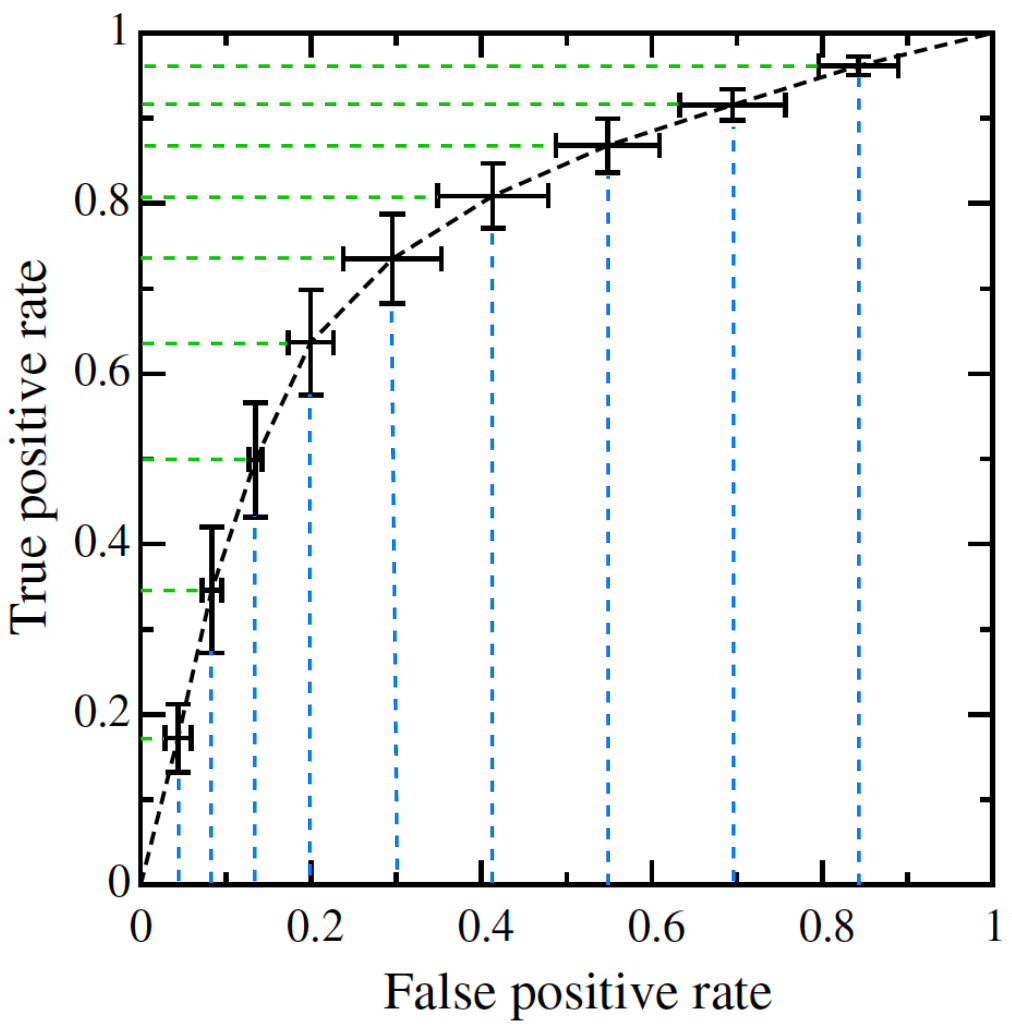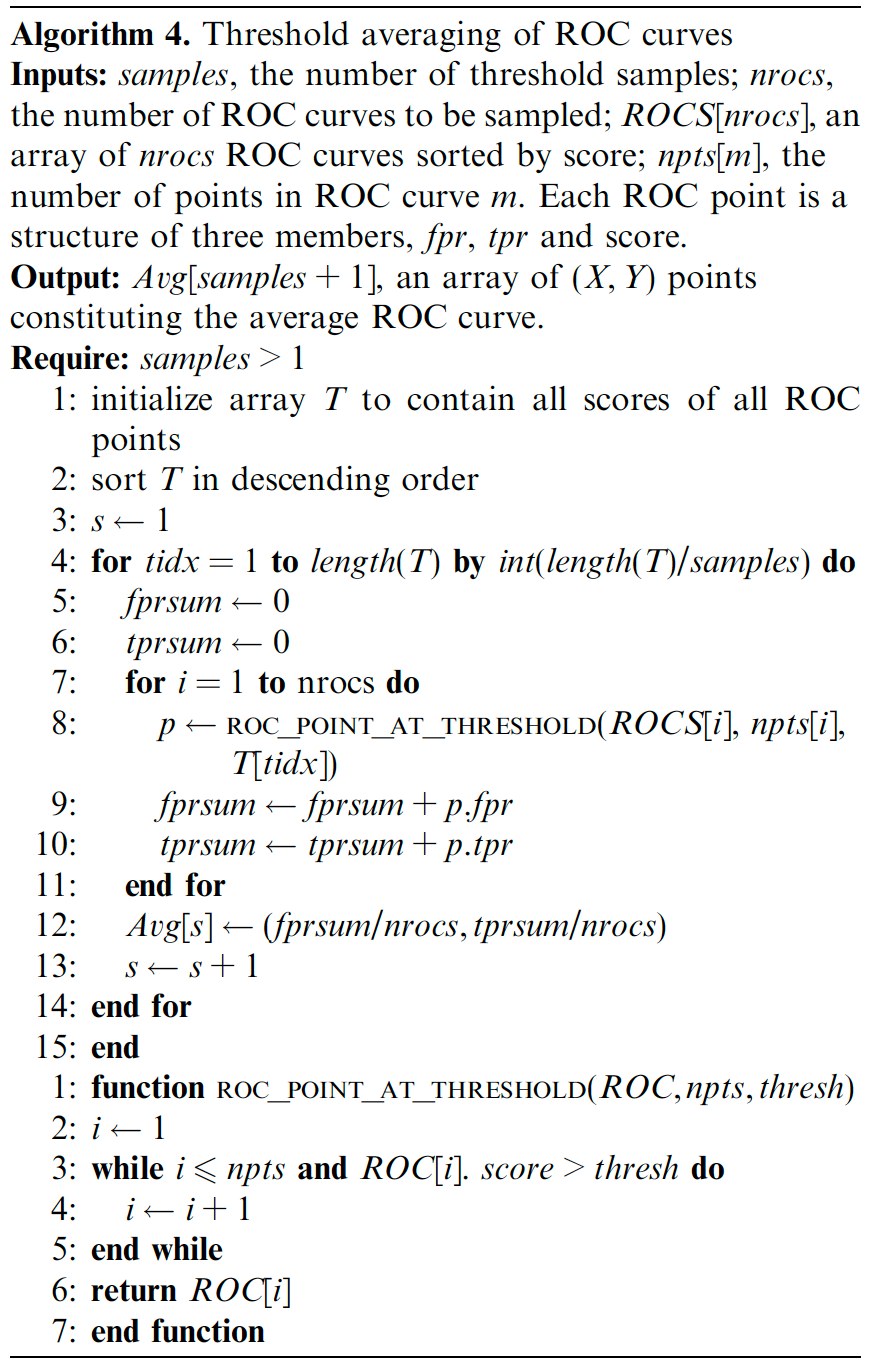# import numpy as np
def roc_threshold_avg(samples, FPR, TPR, THRESHOLDS):
"""
samples：选取FPR点的个数
FPR：包含所有FPR的列表
TPR：包含所有TPR的列表
THRESHOLDS：包含所有THRESHOLDS的列表
"""
nrocs = len(FPR)
T = []
fpravg = []
tpravg = []

for thresholds in THRESHOLDS:
for t in thresholds:
T.append(t)
T.sort(reverse=True)

for tidx in range(0, len(T), int(len(T) / samples)):
fprsum = 0
tprsum = 0
# 将所有计算的fpr和tpr累加
for i in range(nrocs):
fprp, tprp = roc_point_at_threshold(FPR[i], TPR[i], THRESHOLDS[i], T[tidx])
fprsum += fprp
tprsum += tprp
# 计算平均的fpr和tpr
fpravg.append(fprsum / nrocs)
tpravg.append(tprsum / nrocs)

return fpravg, tpravg

# 计算对应threshold的fpr和tpr
def roc_point_at_threshold(fpr, tpr, thresholds, thresh):
i = 0
while i < len(fpr) - 1 and thresholds[i] > thresh:
i += 1
return fpr[i], tpr[i]


## 五、最后

PrimiHub 一款由密码学专家团队打造的开源隐私计算平台，专注于分享数据安全、密码学、联邦学习、同态加密等隐私计算领域的技术和内容。0 评论
2 收藏
0